# Texas Go Math Grade 5 Lesson 17.6 Answer Key Make a Budget

Refer to our Texas Go Math Grade 5 Answer Key Pdf to score good marks in the exams. Test yourself by practicing the problems from Texas Go Math Grade 5 Lesson 17.6 Answer Key Make a Budget.

## Texas Go Math Grade 5 Lesson 17.6 Answer Key Make a Budget

Unlock the problem

A budget is balanced when expenses equal income. Answer the questions, make your choices, and fill in the charts to make and balance a budget.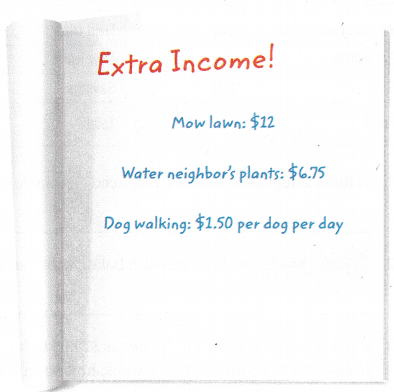Make a budget.

Your allowance is $20 per week. Circle one of these ways to earn extra income. Decide how often you will do it in one week. When you list your expenses, remember to include funds for fun and entertainment, as well as repeating expenses like lunch, bus fare, lessons you pay for, regular donations, savings, and emergency money. A. What is your weekly income?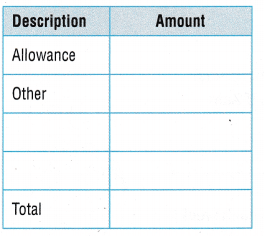Income – expenses = ____ B. What are you weekly expenses?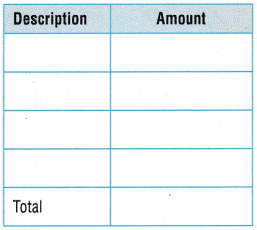Redo your budget, if needed, to make expenses equal income. • You forgot to budget$10 for a birthday gift for your best friend. How can you adjust your budget to fit the new expense?

Share and Show

Complete the tables to find Rob’s total income and total expenses for the week. Use the tables for 1-3.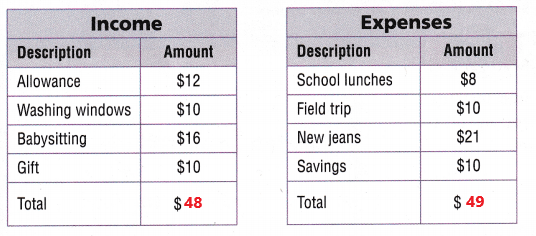Question 1.
Is Rob’s budget for the week balanced? Explain.
No, Rob is spending more money than he is receiving.
Explanation:
In the above two tables we can observe income and expenses. The total income for the week is $48 and the total expenses for the week is$49. Rob expenses is greater than income. Rob’s budget for the week is not balanced because he is is spending more money than he is receiving.

Question 2.
Write Math How can Rob balance the budget?
Rob needs to receive more income. Maybe he can do more babysitting.
Explanation:
To balance the budget Rob needs to receive more income. To receive more amount he has to do more babysitting.

Question 3.
Rob is saving for a tool kit. He needs $79.99 to buy the kit. If he continues to save the same amount each week, how soon will he be able to buy it? Answer: 1 week =$10 each
$10 x 8 weeks =$80
Rob can buy the tool kit after 8 weeks.
Explanation:
In the above expenses chart we can observe Rob is saving $10 for a tool kit. He needs$79.99 to buy the kit. Each week he is saving $10. Multiply$10 with 8 week the product is $80. Rob can buy the tool kit after 8 weeks. Problem Solving Question 4. Analyze For every$10 Zain earns, he will budget $2 for savings,$3 for special purchases, $1 for donating to charity, and$4 for his regular expenses. If Zain earns $200, how much will he budget for his regular expenses? Answer:$200/$10 =$20
$4 x$20 = $80 for regular expenses The budget for his regular expenses are$80.
Explanation:
For every $10 Zain earns, he will budget$2 for savings, $3 for special purchases,$1 for donating to charity, and $4 for his regular expenses. If Zain earns$200 we have to calculate the budget for his regular expenses. First divide $200 y$10 then the result is $20. Multiply$4 with $20 then the product is$80. The budget for his regular expenses are $80. Question 5. H.O.T. Multi-Step Rhonda budgets for the following expenses each week:$5 for bus tickets, $20 for lunch,$10 for movies, and $5 for savings. Rhonda gets$20 as her weekly allowance and $5 for each garden she weeds. If Rhonda’s weekly budget is balanced, how many gardens does she weed each week? Answer: Expenses$5 + $20 +$10 + $5 =$40
Income
$20 +$5 x each garden = $40$20 + $5 x 4 =$40
$20 +$20 = $40 Each week she weed 4 gardens. Explanation: Rhonda budgets for the following expenses each week is$5 for bus tickets, $20 for lunch,$10 for movies, and $5 for savings. Add all the expenses of Rhonda. Add$5 with $20 ,$10 and $5 then the sum is$40. Rhonda total expenses are $40. Rhonda gets$20 as her weekly allowance and $5 for each garden she weeds. The equation is$20 with $5 for each garden is equal to$40. Multiply 45 with 4 gardens then we get $20. Then Rhonda’s weekly budget is balanced. Each week she weed 4 gardens. Question 6. H.O.T. Hazem’s weekly income is$40. He budgets $20 for food and entertainment each week and saves the rest of his income. How much money will he save in 5 months? Answer: Income is$40.
He budgets $20 for food and entertainment each week. He saves$20 for each week.
1 month = 4 weeks
5 months = ? weeks
5months x 4 weeks = 20 weeks
Five months is equal to 20 weeks.
1 week = $20 20 weeks =$?
20 weeks x $20 =$400
He saves $400 for 5 months. Explanation: Hazem’s weekly income is$40. He budgets $20 for food and entertainment each week and saves the rest of his income. Hazem’s saves$20 for each week. We know that there are 4 weeks in one month. Multiply 4 weeks with 5 months then the product is 20 weeks. Multiply 20 weeks with $20 then the result is equal to$400. He saves $400 for 5 months. Problem Solving Question 7. Multi-Step The tables show Diana’s income and expenses for one week. Find the amount for other expenses that will balance her budget for that week.Answer: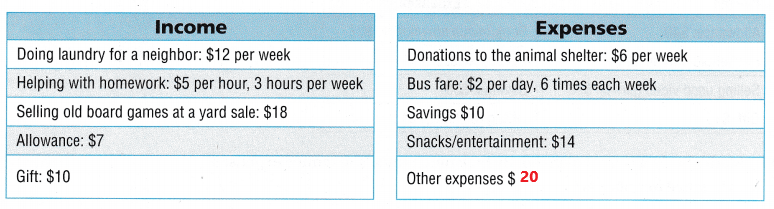Income per week$12 + ($5 x 3) +$18 + $7 +$10 = $62 Expenses per week$6 + ($2 x 6) +$10 + $14 + ? =$62
$42 + ? +$62
$42 +$20 = $62 The other expenses is$20 will balance her budget for that week.
Explanation:
The above tables show Diana’s income and expenses for one week. First add all incomes. Add $12 with$15, $18 ,$7 and $10 the sum is$62. Next add all the expenses. Add $6 with$12, $10,$14, and other expenses the sum must be equal to $62. The other expenses is$20 will balance her budget for that week.

Question 8
H.O.T. Mia earns $15 from babysitting and$9 from dog-walking each week. Her weekly allowance is $15. Mia budgets a third of her income each week for savings and the rest for repeated expenses. How much money does she have in her budget for repeated expenses? ____Answer: Mia’s income$15 + $9 +$15 = $39 (1/3) x$39 = $13 Mia’s saving is$13.
The repeated expenses
$39 –$13 = $26 she have$26 in her budget for repeated expenses.
Explanation:
Mia earns $15 from babysitting and$9 from dog-walking each week. Her weekly allowance is $15. Add all the incomes then the sum is$39. Mia budgets a third of her income each week for savings and the rest for repeated expenses. Multiply (1/3) with $39 the result is$13. Mia’s saving is $13. she have$26 in her budget for repeated expenses.

Question 9.
Record The tables show Han’s monthly budget. Complete the tables to show the total income, total expenses, and the amount of savings if the budget is balanced.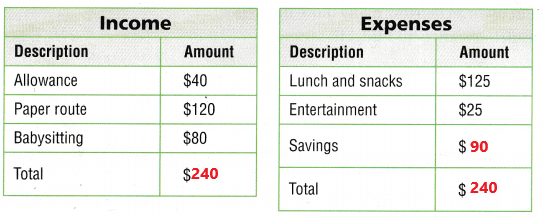The total income
$40 +$120 + $80 =$240
The total expenses
$125 +$25 + Savings = $240 Savings =$240 – $150 =$90
If the budget is balanced the amount of savings is $90. Explanation: The above tables show Han’s monthly budget. To calculate the total income we need to add all earnings. Add$40 with $120 and$80 the sum is $240. The total income is$240. Add the expenses. If the budget is balanced the amount of savings is $90. Question 10. Tanja saves$75 each week. Her other expenses are four times the amount she saves. If Tanja’s weekly budget is balanced, what is her weekly income? _____
Tanja saves $75 each week. Tanja other expenses are four times the amount she saves. 4 x$75 = $300 Tanja’s weekly expenses$300 + $75 =$375
If Tanja’s weekly budget is balanced. Her weekly income is $375. Explanation: Tanja saves$75 each week. Her other expenses are four times the amount she saves. Multiply 4 with $75 the product is$300. Add $300 with$75 the sum is $375. Tanja’s weekly expenses is$375. If Tanja’s weekly budget is balanced. Her weekly income is $375. Question 11. Multi-Step Yoav earns$8 after taxes for every hour he works at the grocery store. He works 15 hours each week at the store. If Yoav budgets a third of his earnings for art supplies, how much money does he have to spend on art supplies each week? _______
1 hour = $8 15 hours =$?
15 x $8 =$120
Yoav income is $120. (1/3) x$120 = $40 He spend$40 on art supplies each week.
Explanation:
Yoav earns $8 after taxes for every hour he works at the grocery store. He works 15 hours each week at the store. Multiply 15 hours with$8 the product is $120. His income is$120 for one week. If Yoav budgets a third of his earnings for art supplies. Multiply (1/3) with $120 the result is$40. He spend $40 on art supplies each week Daily Assessment Task Fill in the bubble completely to show your answer. Use the chart for 12-13.Question 12. Madi’s budget is not balanced. By how much must she reduce expenses to balance her budget? (A)$85
(B) $18 (C)$93
(D) $8 Answer:Income$40 + $20 +$10 + $15 =$85
Expenses
$26 +$22 + $30 +$15 = $93$93 – $85 =$8
She has to reduce expenses $8 to balance her budget. So, option D is correct. Explanation: In the above chart we can observe Madi’s income and expenses. Add all the incomes. Add$40 with $20 ,$10 and $15 the sum is$85. Madi’s total income is $85. Add all the expenses. Add$26 with $22,$30 and $15 the sum is$93. Her total expenses are $93. Subtract income from expenses then the difference is equal to budget balance. Subtract$85 from $93 the difference is$8. She has to reduce expenses $8 to balance her budget. So, option D is correct. Question 13. Madi reduces her monthly expense for book/music downloads to$13. How much more money can she put away for savings and still balance the budget?
(A) $13 (B)$5
(C) $30 (D)$35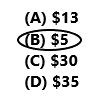Madi reduces her monthly expense for book/music downloads to $13. She reduces$26 to $13.$26 – $13 =$13
Expenses
$93 –$13 = $80 Income is$85.
Savings is a part of expenses, So we can spend $5 more on savings then our expenses will be$85.
So, option B is correct.
Explanation:
Madi reduces her monthly expense for book/music downloads to $13. She reduces$26 to $13. Subtract$13 from $26 the difference is$13. The total expenses are $93 and the total income is$85 as we can observe in the above chart. Subtract $13 from$93 the difference is $80. Savings is a part of expenses, So we can spend$5 more on savings then our expenses will be $85. So, option B is correct. Question 14. Multi-Step Carlos is paid$17 per hour after taxes. He works 25 hours per week. His weekly expenses add up to $476. How many more hours per week would Carlos have to work to balance his budget? (A) 1 hour (B) 3 hours (C) 0 hours (D) 28 hours Answer: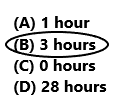1 hour =$17
25 hours = $? 25 x$17 = $425 Carlos income is$425.
His weekly expenses add up to $476.$476 – $425 =$51
$51/$17 = 3 hours
Carlos have to work more 3 hours per week to balance his budget.
So, option B is correct.
Explanation:
Carlos is paid $17 per hour after taxes. He works 25 hours per week. Multiply 25 hours with$17 then the result is $425. Carlos income is$425. His weekly expenses add up to $476. Subtract income from expenses the difference is equal to the budget balance. Subtract$425 from $476 the difference is$51. Here we need to find how many more hours he need to work for the budget balance in both income and expenses. Divide $51 by$17 the result is 3 hours. Carlos have to work more 3 hours per week to balance his budget. So, option B is correct.

Texas Test Prep

Question 15.
Georgette balances her budget by using this plan. For every $100 she earns, she budgets$10 for charity, $20 for savings,$20 for books, and $50 for other expenses. If her income is$500 per week, how much does she give to charity each week?
(A) $500 (B)$50
(C) $5 (D)$250$500/$100 = $5$5 x $10 =$50
She gives $50 to charity each week. So, option B is correct. Explanation: Georgette balances her budget by using this plan. For every$100 she earns, she budgets $10 for charity,$20 for savings, $20 for books, and$50 for other expenses. If her income is $500 per week how much she gives to charity each week. Divide$500 by $100 the result is equal to$5. Multiply $5 with$10 for charity the product is equal to $50. She gives$50 to charity each week. So, option B is correct.

### Texas Go Math Grade 5 Lesson 17.6 Homework and Practice Answer Key

The chart shows Kristi’s January budget. Use the chart for 1-2.

Question 1.
What are Kristi’s total expenses for January?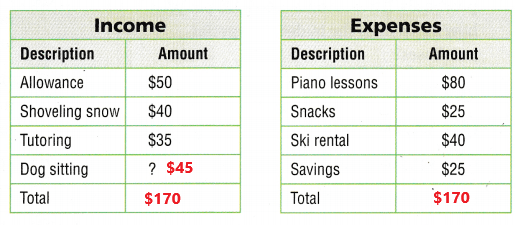Total expenses
$80 +$25 + $40 +$25 = $170 Kristi’s total expenses for January is$170.
Explanation:
The above chart shows Kristi’s January budget. Add all the expenses of Kristi’s from the above chart. Kristi’s total expenses for January is $170. Question 2. How much will Kristi need to earn dog sitting in January for her budget to be balanced? Answer:$50 + $40 +$35 + Dog sitting = $170 Dog sitting =$170 – $125 =$45
Kristi need to earn $45 for dog sitting in January for her budget to be balanced. Explanation: In the above chart we can observe Kristi’s income in January. To calculate dog sitting earning we need to subtract$125 from $170 the difference is$45. Kristi need to earn $45 for dog sitting in January for her budget to be balanced. Question 3. Kristi is saving for a pair of binoculars. The binoculars cost$125.99. If she saves an additional $2 each week, can she buy the binoculars in 5 weeks? Explain. Answer: Kristi is saving$25 for a pair of binoculars.
The binoculars cost $125.99. She saves$25 for pair of binoculars per week.
1 week = $25 5 weeks = ?$25 x 5 = $125 She saves$125 for 5 weeks.
If she saves an additional $2 each week.$2 x 5 weeks = $10$125 + $10 =$135
She can buy a pair of binoculars in 5 weeks.
Explanation:
Kristi is saving $25 for a pair of binoculars. The binoculars cost is$125.99. Multiply 425 with 5 weeks the sum is $125. She saves$125 for 5 weeks. If she saves an additional $2 each week. Multiply$2 with 5 weeks the product is $10. Add$125 with $10 the sum is$135. She saved $135 to buy a pair of binoculars. She can buy a pair of binoculars in 5 weeks. Problem Solving The chart shows Donald’s expenses for one week. Use the chart for 4-5. Question 4. What are Donald’s expenses for the week? Answer: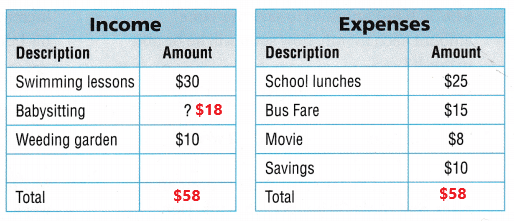Total expenses$25 + $15 +$8 + 410 = $58 Donald’s total expenses for the week is$58.
Explanation:
The above chart shows Donald’s expenses for one week. Add all the expenses. Add $25 with$15, $8 and$10 the sum is $58. Donald’s total expenses for the week is$58.

Question 5.
How much does Donald earn for baby sitting if his weekly budget is balanced?
Income
$30 + baby sitting +$10 = $58 baby sitting =$58 – $40 =$18
Donald earn $18 for baby sitting if his weekly budget is balanced. Explanation: We need to calculate for baby sitting earnings. Subtract$40 from $58 the difference is equal to$18. Donald earn $18 for baby sitting if his weekly budget is balanced. Texas Test Prep Lesson Check Fill in the bubble completely to show your answer. Question 6. Pilar has a plan for her budget. For every$10 she earns, she will budget $4 for savings,$4 for her regular expenses, and $2 for special purchases. If her income is$64,000 per year, how much does she budget for special
purchases?
(A $6,400 (B)$25,600
(C) $12,800 (D)$32,000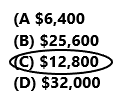$64,000/$10 = $6,400$6,400 x $2 =$12,800
Her budget is $2,800 for special purchases. So, option c is correct. Explanation: Pilar has a plan for her budget. For every$10 she earns, she will budget $4 for savings,$4 for her regular expenses, and $2 for special purchases. If her income is$64,000 per year then we have to calculate the budget for special purchases. Divide $64,000 by$10 the result is equal to $6,400. Multiply$6,400 with $2 the product is$12,800. Her budget is $2,800 for special purchases. So, option c is correct. Question 7. Which statement is true about Mr. Simon’s monthly budget? (A) His budget is balanced when his monthly expenses equal total income for the month. (B) His budget is balanced when total monthly expenses are greater than his income. (C) His budget is balanced when total income is greater than his expenses. (D) His budget is balanced when his weekly expenses are less than his monthly expenses. Answer: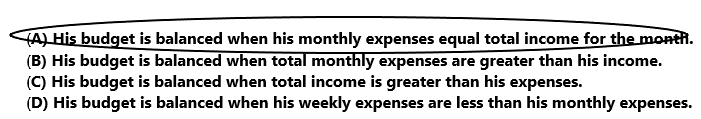Option A is correct. Explanation: Mr. Simon’s monthly budget is balanced when his monthly expenses is equal to total income for the month. So, option A is correct. The chart shows Ellison’s April budget. Use the chart for 8-9.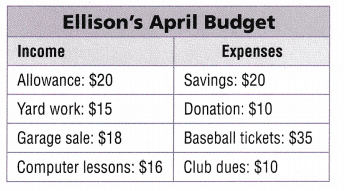Question 8. Ellison is saving for a skateboard that costs$49.75. If he continues to save the same amount each month, how soon will he be able to buy it?
(A) 3 months
(B) 2 months
(C) 3 weeks
(D) 2 weeks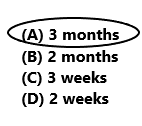Ellison is saving for a skateboard that costs $49.75.$20 x 3 months = $60 He will be able to buy a skateboard in 3 months. So, Option A is correct. Explanation: Ellison is saving for a skateboard that costs$49.75. In one month he saved $20. Multiply$20 with 3 months the result is $60. He will be able to buy a skateboard in 3 months. So, Option A is correct. Question 10. Multi-Step Shelley earns$12 per hour after taxes. She works 30 hours each week. Her weekly expenses are $420. How many more hours per week would she have to work to balance her budget? (A) 6 hours (B) 5 hours (C) 2 hours (D) 3 hours Answer: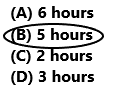Shelley’s income 1 hour =$12
30 hours = $?$12 x 30 hours = $360 Shelly’s income for a week is$360.
Her weekly expenses are $420.$420 – $360 =$60
$12 x 5 hours =$60
She have to work 5 more hours to balance her budget.
So, option B is correct.
Explanation:
Shelley earns $12 per hour after taxes. She works 30 hours each week. Multiply$12 with 30 hours the product is equal to $360. Shelly’s income for a week is$360. Her weekly expenses are $420. Subtract$360 from $420 the difference is$60. Multiplying $12 with 5 hours the product is equal to$60. She have to work 5 more hours to balance her budget. So, option B is correct.

Question 11.
Multi-Step Tyrone earns $650 per week. This week, Tyrone wants to donate$100 to charity. His weekly expenses are $595. How much will Tyrone need to reduce his expenses in order to make the donation and balance his budget? (A)$55
(B) $40 (C)$50
(D) $45 Answer: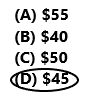Tyrone earns$650 per week.
$100 +$595 = $695 Tyrone total expenses is$695.
$695 –$650 = $45 Tyrone needs to reduce his expenses$45 in order to make the donation and balance the budget.
So, option D is correct.
Explanation:
Tyrone earns $650 per week. This week, Tyrone wants to donate$100 to charity. His weekly expenses are $595. Add$100 with $595 the sum is$695. Tyrone total expenses is $695. Subtract income$650 from expenses $695 the difference is$45. Tyrone needs to reduce his expenses \$45 in order to make the donation and balance the budget. So, option D is correct.

Scroll to Top